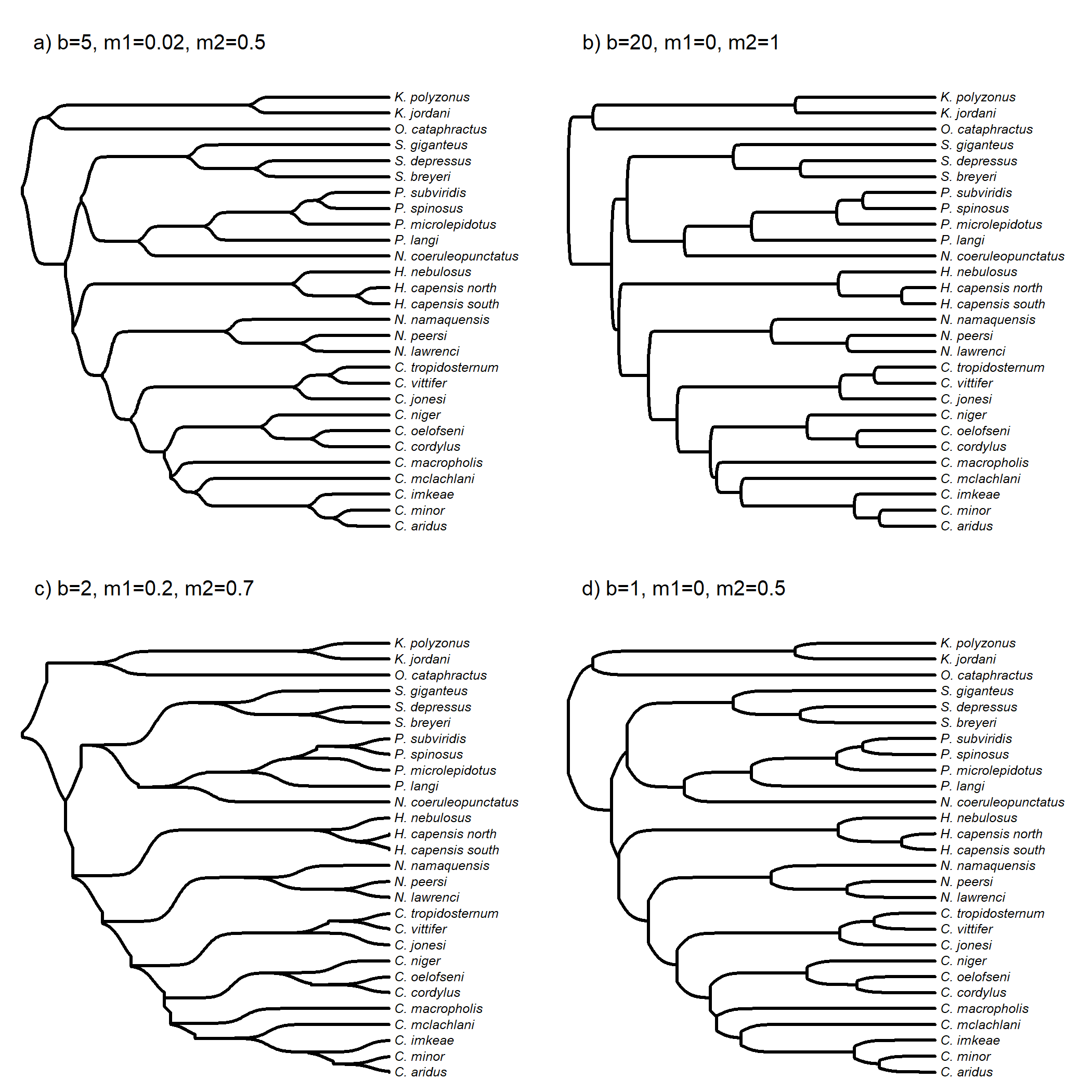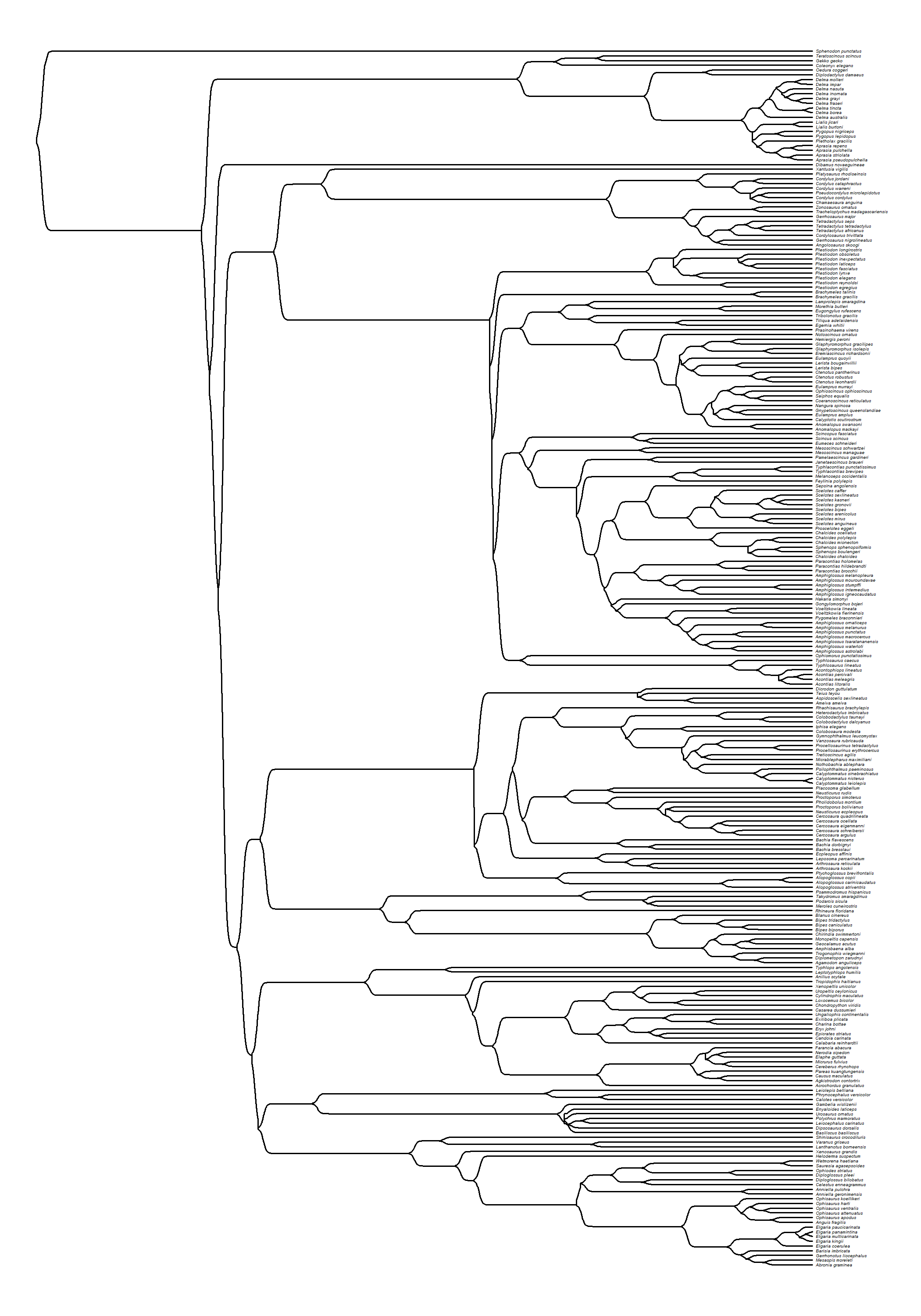## Friday, August 5, 2022

### Optional function arguments (and their effects) in `phytools::sigmoidPhylogram`

Yesterday I blogged about a new phytools function, `sigmoidPhylogram`, to plot the branches of a phylogram or cladogram in sigmoidal style.

I use the generalized logistic function, but the majority of function arguments have values beyond the control of the user. (For the most part, this is because it wouldn't make sense to specify values different from the default.)

I've left a few to be specified, although I tried to set reasonable defaults to get the kind of plot that I was going for with the function! In particular, the argument `b` (which defaults to `5`), controls the rate of growth of the logistic function (higher values grow more steeply); `v`, determines how the asymptote is approached; and `m1` & `m2`, the other two arguments that the user can set, determine how close the start (`m1`) and the end (`m2`) of the sigmoid are to the beginning (`0`) and end (`1`) of the edge.

To see how these different arguments affect our plot, here I'll adjust only `b`, `m1`, and `m2`.

For this example, I'll use a phylogeny of cordylid lizards from Broeckhoven et al. (2016).

``````library(phytools)
par(mfrow=c(2,2))
sigmoidPhylogram(cordylid.tree,fsize=0.6,ftype="i",lwd=2,
m1=0.02,mar=c(0.1,0.1,3.1,0.1))
cex=0.8)
sigmoidPhylogram(cordylid.tree,fsize=0.6,ftype="i",lwd=2,
b=20,m1=0,m2=1,mar=c(0.1,0.1,3.1,0.1))
cex=0.8)
sigmoidPhylogram(cordylid.tree,fsize=0.6,ftype="i",lwd=2,
b=2,m1=0.2,m2=0.7,mar=c(0.1,0.1,3.1,0.1))
cex=0.8)
sigmoidPhylogram(cordylid.tree,fsize=0.6,ftype="i",lwd=2,
b=2,m1=0,m2=0.5,mar=c(0.1,0.1,3.1,0.1))
cex=0.8)
``````On top of that, for fun here's a substantially larger tree of squamate reptiles.

This tree comes from Brandley et al. (2008).

``````squamate.tree<-read.nexus(file="http://www.phytools.org/Rbook/11/squamate.tre")
sigmoidPhylogram(squamate.tree,ftype="i",fsize=0.2,lwd=1,b=2,m1=0.007)
``````Neat.